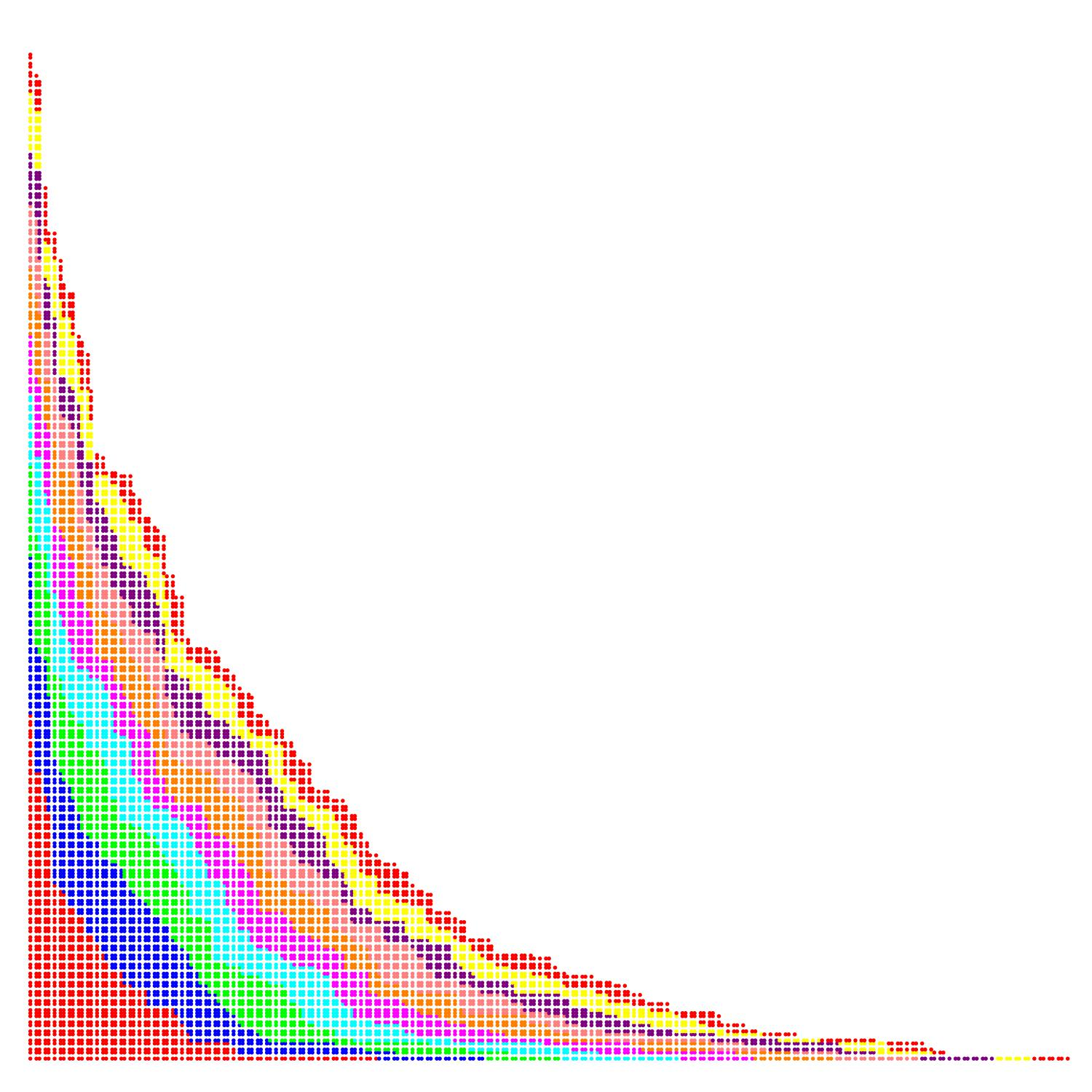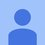# Grid and ProbabilityConsider the first quadrant of a $2D$ Cartesian system as a grid. A recursive function "marks" solely the boxes in the grid (one at a time) that neighbor exactly two other marked ones. Such boxes are referred to as "kink sites". The x and y axes are assumed to be already marked as an initial condition. At each iteration, all kink sites have equal probability of getting marked. So, e.g. starting with the unmarked grid the first and only box that can be marked is the one adjacent to the corner of the grid (box $(1,1)$). Subsequently, there are two options; either box $(1,2)$ above, or box $(2,1)$ to the right of the marked corner box, both equally likely to be marked with probability $0.5$. The following kink sites keep getting marked in the same manner. The attached image (grid not shown) shows all marked boxes after $10,000$ computer simulated iterations. Different colors represent any bunch of $1,000$ iterations. As the number of marked boxes grows infinitely, if any, what algebraic function is traced by the kink site front?Note by Tomasz Bukowy
1 year, 4 months ago

This discussion board is a place to discuss our Daily Challenges and the math and science related to those challenges. Explanations are more than just a solution — they should explain the steps and thinking strategies that you used to obtain the solution. Comments should further the discussion of math and science.

When posting on Brilliant:

• Use the emojis to react to an explanation, whether you're congratulating a job well done , or just really confused .
• Ask specific questions about the challenge or the steps in somebody's explanation. Well-posed questions can add a lot to the discussion, but posting "I don't understand!" doesn't help anyone.
• Try to contribute something new to the discussion, whether it is an extension, generalization or other idea related to the challenge.

MarkdownAppears as
*italics* or _italics_ italics
**bold** or __bold__ bold
- bulleted- list
• bulleted
• list
1. numbered2. list
1. numbered
2. list
Note: you must add a full line of space before and after lists for them to show up correctly
paragraph 1paragraph 2

paragraph 1

paragraph 2

[example link](https://brilliant.org)example link
> This is a quote
This is a quote
    # I indented these lines
# 4 spaces, and now they show
# up as a code block.

print "hello world"
# I indented these lines
# 4 spaces, and now they show
# up as a code block.

print "hello world"
MathAppears as
Remember to wrap math in $$ ... $$ or $ ... $ to ensure proper formatting.
2 \times 3 $2 \times 3$
2^{34} $2^{34}$
a_{i-1} $a_{i-1}$
\frac{2}{3} $\frac{2}{3}$
\sqrt{2} $\sqrt{2}$
\sum_{i=1}^3 $\sum_{i=1}^3$
\sin \theta $\sin \theta$
\boxed{123} $\boxed{123}$

Sort by:

Grid is something is like a pattern of straight lines that can are a diagonal cross over each other, it is formatting like squares. this is placed in particular things or places. this is a graphics design that is a series of vertical and horizontal lines. probability is the likelihood of an event that occurs it is something which is certain to have is 0 or 1 there bis only true-false condition. this graph is most widely used by the Cover letter writers because to check the improvement in their own skills.

- 10 months, 1 week ago